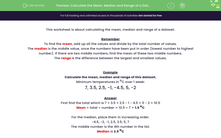# Calculate the Mean, Median and Range of a Dataset (2)

In this worksheet, students must calculate the mean, median and range of a dataset.Key stage:  KS 3

Curriculum topic:   Statistics

Curriculum subtopic:   Understand Variables, Representation, Measures and Spread

Difficulty level:#### Worksheet Overview

This worksheet is about calculating the mean, median and range of a dataset.

Remember

To find the mean, add up all the values and divide by the total number of values.

The median is the middle value, once the numbers have been put in order (lowest number to highest number). If there are two middle numbers, find the mean of these two middle numbers.

The range is the difference between the largest and smallest values.

Example

Calculate the mean, median and range of this dataset.

Minimum temperatures in oC over 1 week:

7, 3.5, 2.5, -1, -4.5, 5, -2

First find the total which is 7 + 3.5 + 2.5 - 1 - 4.5 + 5 - 2 = 10.5

Mean = total ÷ number = 10.5 ÷ 7 = 1.5 oC

For the median, place them in increasing order.

-4.5, -2, -1, 2.5, 3.5, 5, 7

The middle number is the 4th number in the list.

Median is 2.5 oC

Range = largest value - smallest value = 7 - -4.5 = 7 + 4.5 = 11 oC

### What is EdPlace?

We're your National Curriculum aligned online education content provider helping each child succeed in English, maths and science from year 1 to GCSE. With an EdPlace account you’ll be able to track and measure progress, helping each child achieve their best. We build confidence and attainment by personalising each child’s learning at a level that suits them.

Get started